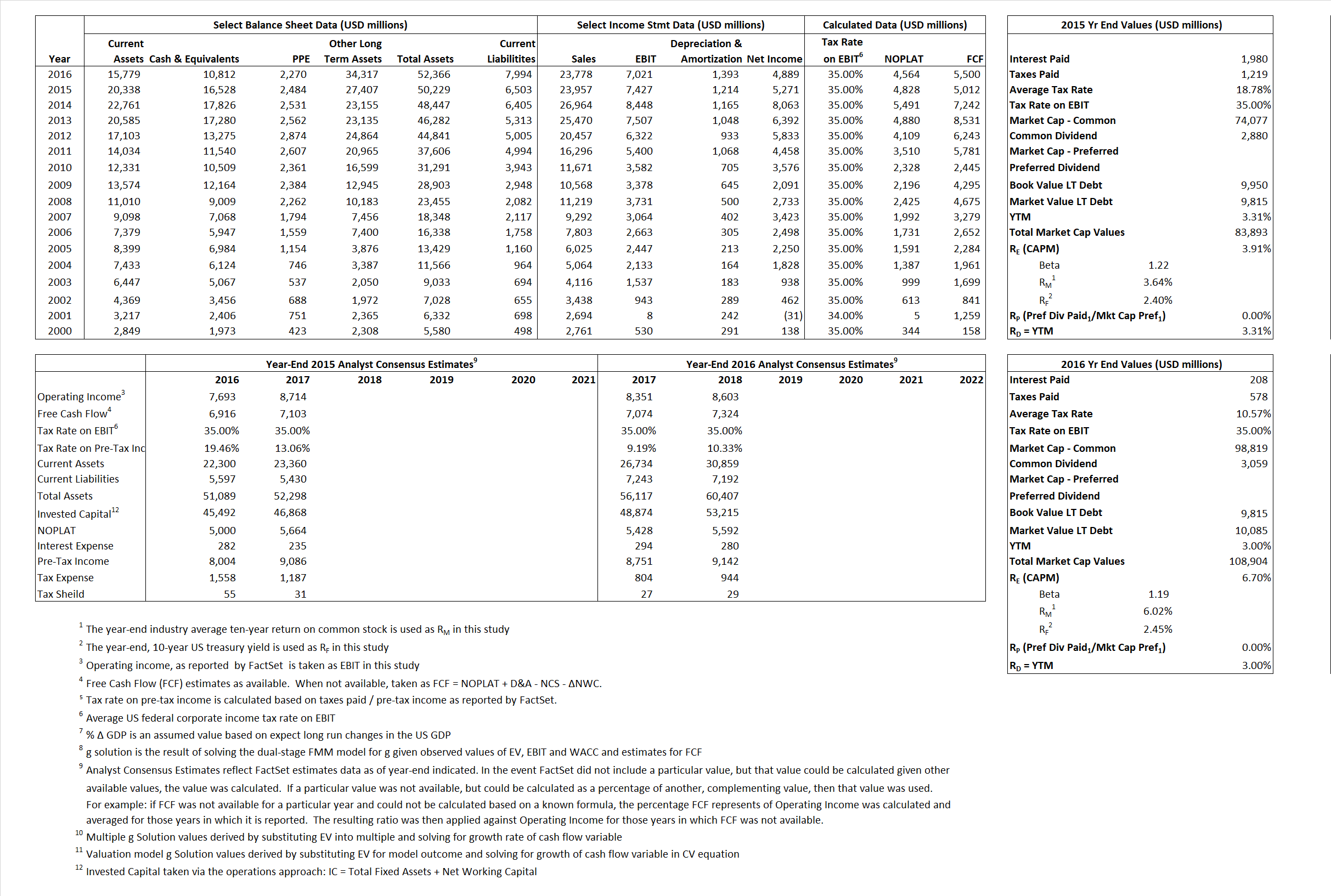# Qualcomm Inc.

## Analyst Listing

The following analysts provide coverage for the subject firm as of May 2016:

 Broker Analyst Analyst Email RBC Capital Markets Amit Daryanani amit.daryanani@rbccm.com William Blair Anil Doradla adoradla@williamblair.com Evercore ISI C.J. Muse cj.muse@evercoreisi.com Atlantic Equities James Cordwell j.cordwell@atlantic-equities.com Stifel Nicolaus Kevin E Cassidy kcassidy@stifel.com Credit Suisse Kulbinder Garcha kulbinder.garcha@credit-suisse.com Pacific Crest Securities-KBCM Michael McConnell mmcconnell@pacific-crest.com Brean Capital, LLC Mike Burton mburton@breancapital.com Oppenheimer Rick Schafer rick.schafer@opco.com Nomura Research Romit Shah romit.shah@nomura.com Bernstein Research Stacy A Rasgon stacy.rasgon@bernstein.com Topeka Capital Markets Suji De Silva sd@topekacapitalmarkets.com Canaccord Genuity T. Michael Walkley mwalkley@canaccordgenuity.com Raymond James Tavis C. McCourt tavis.mccourt@raymondjames.com Northland Securities Thomas Sepenzis tsepenzis@northlandcapitalmarkets.com BMO Capital Markets Tim Long tim.long@bmo.com Cowen & Company Timothy Arcuri timothy.arcuri@cowen.com Mizuho Securities USA Vijay Rakesh vijay.rakesh@us.mizuho-sc.com Daiwa Securities Co. Ltd. Yoko Yamada yoko.yamada@us.daiwacm.com

## Primary Input Data## Derived Input Data

### Equational Form

Net Operating Profit Less Adjusted Taxes NOPLAT  4,828 4,564$NOPLAT\, =\, EBIT\, x\, (1 \,-\, Avg \,\,Tax\,\, Rate\,\, on\,\, EBIT)$
Free Cash Flow FCF 5,012 5,500$FCF\,=NOPLAT\,+\,Non-Cash\,Expenses-\Delta NWC\,-\,NCS$
Tax Shield TS 372 22$TS\,=\,Interest\,\,Paid\,\,x\,\, Avg \,\,Tax\,\,Rate\,\, on\,\, Pre-Tax\,\, Income$
Invested Capital IC 43,726 44,372$IC\,=\,Fixed\,\,Operating\,\,Assets\,\,+\,\,Net\,\, Working\,\, Capital$
Return on Invested Capital ROIC 11.04% 10.28%$ROIC\,=\,\frac { NOPLAT }{ IC }$
Net Investment NetInv 2,898 2,039$NetInv\,=\,{ {IC}_{1}}-{{IC}_{0}}+Depreciation$
Investment Rate IR  60.03% 44.68%$IR\,=\,\frac {NetInv}{NOPLAT}$
Weighted Average Cost of Capital
WACCMarket 3.77% 6.33%$WACC\,=\,\frac { E }{ V } { R }_{ E }\,+\,\frac { P }{ V } { R }_{ P }\,+\,\frac { D }{ V } { R }_{ D }\left( 1- Avg\,\, Tax\,\,Rate\,\,on\,\,Pre-Tax\,\,Income \right)$
WACCBook  9.60%  9.34%
Enterprise value
EVMarket 67,365 98,092$EV\,=\,Market\,\,Cap\,\,Equity\,+\,\,Long\,\,Term\,\,Debt\,-\,Cash$
EVBook   73,215  97,822
Long-Run Growth
g = IR x ROIC
6.63%  4.60% Long-run growth rates of the income variable are used in the Continuing Value portion of the valuation models.
g = %$\Delta$ GDP   2.50%   2.50%
Margin from Operations M  31.00%  29.53%$M\,\,=\,\,\frac{EBIT}{SALES}$
Depreciation/Amortization Rate D  14.05%  16.56%$D\,\,=\,\,\frac{D+A}{EBITDA}$

## Valuation Multiple Outcomes

The outcomes presented in this study are the result of original input data, derived data, and synthesized inputs.

### model g solution

12/31/2015 12/31/2016 12/31/2015 12/31/2016 12/31/2015 12/31/2016

EV/SALES$\frac {EV}{Sales} \,= \,\frac{ROIC\, -\, g}{ROIC\,(WACC\,-\,g)}\,(1\,-\,T)\,(M)$

2.81  4.13  -9.68%  3.06%  -18.11%  3.69%

EV/EBITDA$\frac {EV}{EBITDA} \,= \,\frac{ROIC\, -\, g}{ROIC\,(WACC\,-\,g)}\,(1\,-\,T)\,(1\,-\,D)$

7.80 11.66 -9.68% 3.06% -18.11% 3.69%

EV/NOPLAT$\frac {EV}{NOPLAT} \,= \,\frac{ROIC\, -\, g}{ROIC\,(WACC\,-\,g)}$

13.95 21.49 -9.68% 3.06% -18.11% 3.69%

EV/FCFOPS$\frac {EV}{FCF_{OPS}} \,= \,\frac{ROIC\, -\, g}{ROIC\,(WACC\,-\,g)}\,(1\,-\,T)$

13.44 17.83 -9.68% 3.06% -18.11% 3.69%

EV/EBIT$\frac {EV}{EBIT} \,= \,\frac{ROIC\, -\, g}{ROIC\,(WACC\,-\,g)}\,(1\,-\,T)$

9.07 13.97 -9.68% 3.06% -18.11% 3.69%

EV/IC$\frac {EV}{IC} \,= \,\frac{ROIC\, -\, g}{WACC\,-\,g}$

1.54 2.21 -9.68% 3.06% -18.11% 3.69%